## Parseval's Theorem

Letbe a continuous function andandbe Fourier Transform pairs so that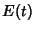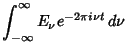(1)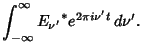(2)

Then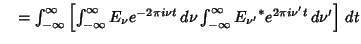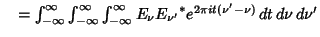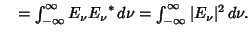(3)
whereis the Delta Function.

For finite Fourier Transform pairsand,(4)

If a function has a Fourier Series given by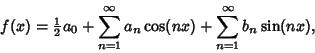(5)

then Bessel's Inequality becomes an equality known as Parseval's theorem. From (5),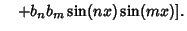(6)
Integrating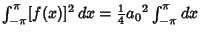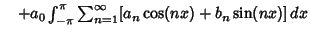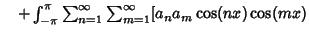(7)
so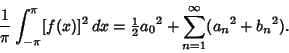(8)

For a generalized Fourier Series with a Complete Basis, an analogous relationship holds. For a Complex Fourier Series,(9)

References

Gradshteyn, I. S. and Ryzhik, I. M. Tables of Integrals, Series, and Products, 5th ed. San Diego, CA: Academic Press, p. 1101, 1979.